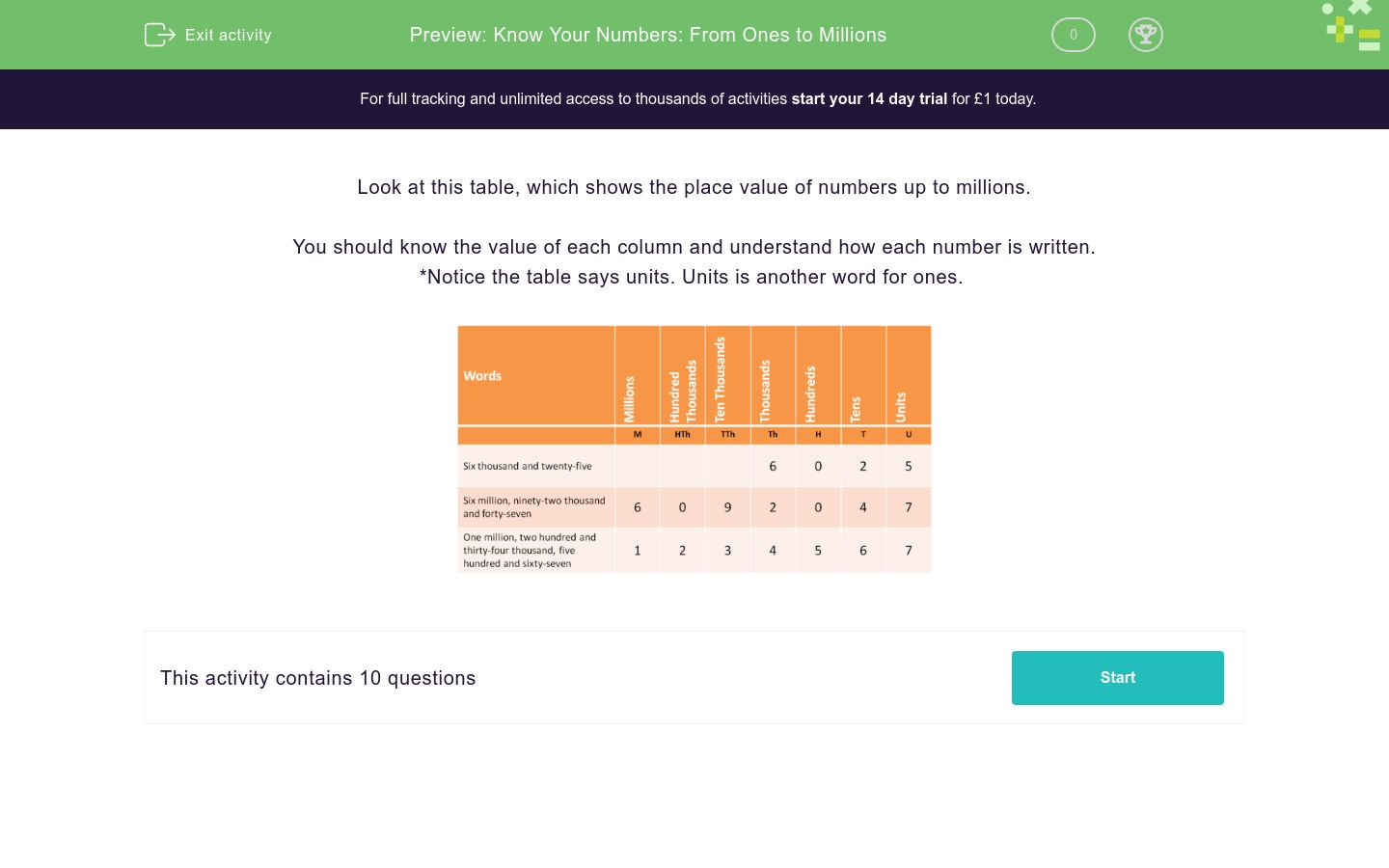# Know Your Numbers: From Ones to Millions

In this worksheet, students read numbers from ones to millions and state the value of the given digits.Key stage:  KS 2

Curriculum topic:  Number: Number and Place Value

Curriculum subtopic:  Determine Digit Value to 10 000 000

Difficulty level:### QUESTION 1 of 10

Look at this table, which shows the place value of numbers up to millions.

You should know the value of each column and understand how each number is written.

*Notice the table says units. Units is another word for ones.When you write the following number using digits, select the column in which you would place the digit  3.

Fifty-three thousand and twenty-six

Millions

Hundred Thousands

Ten Thousands

Thousands

Hundreds

Tens

UnitsWhen you write the following number using digits, select the column in which you would place the digit  3.

Fifty-two thousand and thirty-six

Millions

Hundred Thousands

Ten Thousands

Thousands

Hundreds

Tens

UnitsWhen you write the following number using digits, select the column in which you would place the digit  3.

Three million, fifty-two thousand and ninety-six

Millions

Hundred Thousands

Ten Thousands

Thousands

Hundreds

Tens

UnitsWhen you write the following number using digits, select the column in which you would place the digit  3.

Five million, three hundred and fifty-two thousand and ninety-six

Millions

Hundred Thousands

Ten Thousands

Thousands

Hundreds

Tens

UnitsWhen you write the following number using digits, select the column in which you would place the digit  3.

Five million, six hundred and fifty-two thousand, three hundred and ninety-six

Millions

Hundred Thousands

Ten Thousands

Thousands

Hundreds

Tens

UnitsWhen you write the following number using digits, select the column in which you would place the digit  3.

Five million, six hundred and fifty-three thousand, seven hundred and ninety-six

Millions

Hundred Thousands

Ten Thousands

Thousands

Hundreds

Tens

UnitsWhen you write the following number using digits, select the column in which you would place the digit  3.

Five million, six hundred and fifty-five thousand, seven hundred and ninety-three

Millions

Hundred Thousands

Ten Thousands

Thousands

Hundreds

Tens

UnitsWhen you write the following number using digits, select the column in which you would place the digit  3.

Five million, three hundred and fifty-five thousand, seven hundred and ninety

Millions

Hundred Thousands

Ten Thousands

Thousands

Hundreds

Tens

UnitsWhen you write the following number using digits, select the column in which you would place the digit  3.

Five million, eight hundred and thirty-five thousand, seven hundred and forty

Millions

Hundred Thousands

Ten Thousands

Thousands

Hundreds

Tens

UnitsWhen you write the following number using digits, select the column in which you would place the digit  3.

Five million, eight hundred thousand and thirty

Millions

Hundred Thousands

Ten Thousands

Thousands

Hundreds

Tens

Units

• Question 1When you write the following number using digits, select the column in which you would place the digit  3.

Fifty-three thousand and twenty-six

Thousands
EDDIE SAYS
53026 - The 3 is in the thousands place.
• Question 2When you write the following number using digits, select the column in which you would place the digit  3.

Fifty-two thousand and thirty-six

Tens
EDDIE SAYS
52036 - The three is in the tens place.
• Question 3When you write the following number using digits, select the column in which you would place the digit  3.

Three million, fifty-two thousand and ninety-six

Millions
EDDIE SAYS
3,052,096 - The 3 is in the millions place.
• Question 4When you write the following number using digits, select the column in which you would place the digit  3.

Five million, three hundred and fifty-two thousand and ninety-six

Hundred Thousands
EDDIE SAYS
5, 352, 096 - The 3 is in the hundred thousands place.
• Question 5When you write the following number using digits, select the column in which you would place the digit  3.

Five million, six hundred and fifty-two thousand, three hundred and ninety-six

Hundreds
EDDIE SAYS
5, 652, 396 - The 3 is in the hundreds place.
• Question 6When you write the following number using digits, select the column in which you would place the digit  3.

Five million, six hundred and fifty-three thousand, seven hundred and ninety-six

Thousands
EDDIE SAYS
5, 653, 796 - The 3 is in the thousands place.
• Question 7When you write the following number using digits, select the column in which you would place the digit  3.

Five million, six hundred and fifty-five thousand, seven hundred and ninety-three

Units
EDDIE SAYS
5, 655, 793 - The three is in the ones place.
• Question 8When you write the following number using digits, select the column in which you would place the digit  3.

Five million, three hundred and fifty-five thousand, seven hundred and ninety

Hundred Thousands
EDDIE SAYS
5, 355, 790 - The three is in the hundred thousands place.
• Question 9When you write the following number using digits, select the column in which you would place the digit  3.

Five million, eight hundred and thirty-five thousand, seven hundred and forty

Ten Thousands
EDDIE SAYS
5, 835, 740 - The 3 is in the ten thousands place.
• Question 10When you write the following number using digits, select the column in which you would place the digit  3.

Five million, eight hundred thousand and thirty

Tens
EDDIE SAYS
5, 800, 030 - The 3 is in the tens place.
---- OR ----

Sign up for a £1 trial so you can track and measure your child's progress on this activity.

### What is EdPlace?

We're your National Curriculum aligned online education content provider helping each child succeed in English, maths and science from year 1 to GCSE. With an EdPlace account you’ll be able to track and measure progress, helping each child achieve their best. We build confidence and attainment by personalising each child’s learning at a level that suits them.

Get startedStart your £1 trial today.
Subscribe from £10/month.